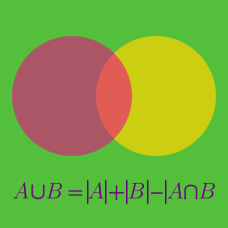Probability

# Principle of Inclusion and Exclusion - Two Sets

Two standard six-sided dice, one red and one blue, are rolled. How many different rolls are there in which at least one dice shows a $5$?

If $A$ and $B$ are two sets such that $\lvert{A \cup B}\rvert=45, \lvert{A \cap B}\rvert=21 \text{ and } \lvert{A \setminus B}\rvert=11,$ then what is the value of $\lvert{B}\rvert?$

Details and assumptions

You may choose to read the summary page Set Notation.

Calvin has 23 books on his shelf, all of which are either Mathematics books or hardcover books. If 18 of the 23 are hardcover books, and 12 of the 23 are Mathematics books, how many hardcover Mathematics books does Calvin have on his shelf?

Details and assumptions

You may choose to read Principle of Inclusion and Exclusion.

If set $A$ contains $57$ elements, set $B$ contains $316$ elements and $A \cap B$ contains $15$ elements, how many elements are contained in $A \cup B?$

Details and assumptions

You may choose to read the summary page Set Notation.

In a group of $52$ students, $38$ are taking physics and $47$ are taking chemistry. If $x$ is the number of students taking both physics and chemistry, then the possible range of $x$ is $a \leq x \leq b.$ What is $b-a?$

×